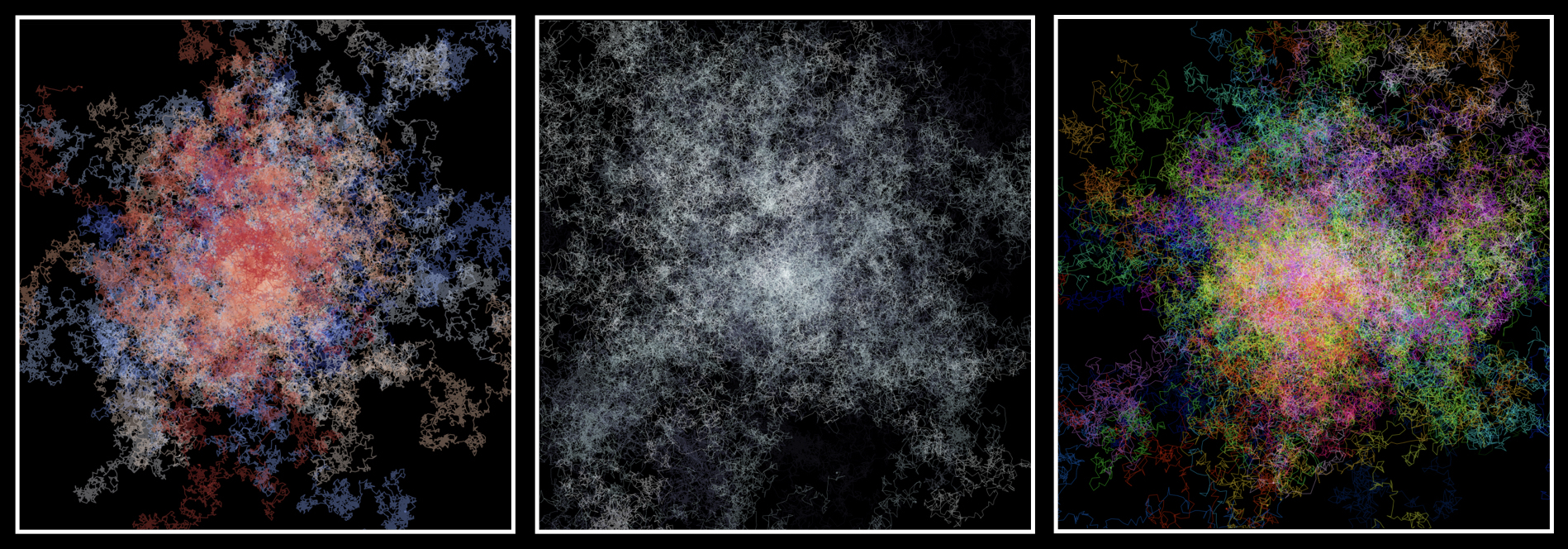## Random walk (art)Trying to kill some time on a 4-hour long train ride I played around with simulating random walk in two dimensions. Coloring each walker with it’s own unique colors, the motion of individual walkers will more or less look like confused ants moving around on a piece of paper. Resembling the behavior illustrated below – see code below.### Code

As usual lets first import some packages. Note, I still use Python 2.7.

import matplotlib.pyplot as plt
import numpy as np


Then we define some parameters, such as how many steps the simulation should run for as well as how many walkers we want to have. All walkers start from the same initial condition, coordinates (0,0), and for each step we randomly select the direction walkers should move in. Last we pick the step length, i.e. how far a walker should move in this given step. Here you can play around by sampling step-length from different distributions, see more below.

# define some parameters
steps = 1000
walkers = 100
x = np.zeros((steps,walkers)); y = np.zeros((steps,walkers))

# go through steps
for step in range(1,steps):

# pick direction
angle = np.random.rand(walkers)*2*np.pi

# pick step length
length = np.random.rand(walkers)
#length = np.random.exponential(size=walkers)
#length = np.random.poisson(lam=2,size=walkers)
#length = np.random.power(a=3,size=walkers)

# update position
x[step] = x[step-1,:]+np.cos(angle)*length
y[step] = y[step-1,:]+np.sin(angle)*length


After having run the simulation (its relatively fast) I use matplotlib to plot the trajectories.

# define plot limits
lim = 30

# define colors - give each walker its own colors
colors = plt.cm.gist_ncar(np.linspace(0,1,walkers))

# plot stuff
plt.figure(figsize=(10,10))
for walker in range(walkers):
plt.plot(x[walker],y[walker],alpha=0.4,color=colors[walker],lw=0.8)

plt.xlim(-lim,lim)
plt.ylim(-lim,lim)

plt.axis('off')